# 7.4 - Mathematical Induction

## The need for proof

Most people today are lazy. We watch way too much television and are content to accept things as true without question.

If we see something that works a few times in a row, we're convinced that it works forever.

### Regions of a Circle

Consider a circle with n points on it. How many regions will the circle be divided into if each pair of points is connected with a chord?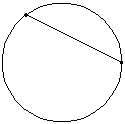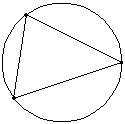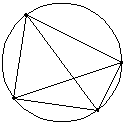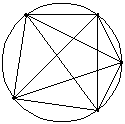2 points 2 regions = 21 3 points 4 regions = 22 4 points 8 regions = 23 5 points 16 regions = 24

At this point, probably everyone would be convinced that with 6 points there would be 32 regions, but it's not proved, it's just conjectured. The conjecture is that the number of regions when n points are connected is 2n -1.

Will finding the number of regions when there are six points on the circle prove the conjecture? No. If there are indeed 32 regions, all you have done is shown another example to support your conjecture. If there aren't 32 regions, then you have proved the conjecture wrong. In fact, if you go ahead and try the circle with six points on it, you'll find out that there aren't 32 regions.

You can never prove a conjecture is true by example.

You can prove a conjecture is false by finding a counter-example.

To prove a conjecture is true, you need some more formal methods of proof. One of these methods is the principle of mathematical induction.

## Principle of Mathematical Induction (English)

1. Show something works the first time.
2. Assume that it works for this time,
3. Show it will work for the next time.
4. Conclusion, it works all the time

## Principle of Mathematical Induction (Mathematics)

1. Show true for n = 1
2. Assume true for n = k
3. Show true for n = k + 1
4. Conclusion: Statement is true for all n >= 1

The key word in step 2 is assume. You are not trying to prove it's true for n = k, you're going to accept on faith that it is, and show it's true for the next number, n = k + 1. If it later turns out that you get a contradiction, then the assumption was wrong.

## Annotated Example of Mathematical Induction

Prove 1 + 4 + 9 + ... + n2 = n (n + 1) (2n + 1) / 6 for all positive integers n.

Another way to write "for every positive integer n" is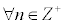. This works because Z is the set of integers, so Z+ is the set of positive integers. The upside down A is the symbol for "for all" or "for every" or "for each" and the symbol that looks like a weird e is the "element of" symbol. So technically, the statement is saying "for every n that is an element of the positive integers", but it's easier to say "for every positive integer n".

Identify the general term and nth partial sum before beginning the problem

The general term, an, is the last term on the left hand side. an = n2
The nth partial sum, Sn, is the right hand side. Sn = n (n + 1) (2n + 1) / 6

Find the next term in the general sequence and the series

The next term in the sequence is ak+1 and is found by replacing n with k+1 in the general term of the sequence, an.

ak+1 = ( k + 1 )2

The next term in the series is Sk+1 and is found by replacing n with k+1 in the nth partial sum, Sn. You may wish to simplify the next partial sum, Sk+1
Sk+1 = (k+1) [ (k+1) + 1 ] [ 2(k+1) + 1 ] / 6
Sk+1 = ( k + 1 ) ( k + 2 ) ( 2k +3 ) / 6 (This will be our Goal in step 3)

We will use these definitions later in the mathematical induction process. We're now ready to begin.

### 1. Show the statement is true for n = 1, that is, Show that a1 = S1.

a1 is the first term on the left or you can find it by substituting n=1 into the formula for the general term, an.
S1 is found by substituting n=1 into the formula for the nth partial sum, Sn.

lhs: a1 = 1
rhs: S1 = 1 ( 1+1 ) [ 2(1) + 1 ] / 6 = 1(2)(3) / 6 = 1

So, you can see that the left hand side equals the right hand side for the first term, so we have established the first condition of mathematical induction.

### 2. Assume the statement is true for n = k

The left hand side is the sum of the first k terms, so we can write that as Sk. The right hand side is found by substituting n=k into the Sn formula.

Assume that Sk = k ( k + 1 ) ( 2k + 1 ) / 6

### 3. Show the statement is true for n = k+1

What we are trying to show is that Sk+1 = ( k + 1 ) ( k + 2 ) ( 2k +3 ) / 6. This was our goal from earlier.

We begin with something that we know (assume) is true and add the next term, ak+1, to both sides.

Sk + ak+1 = k ( k + 1 ) ( 2k + 1 ) / 6 + ak+1

On the left hand side, Sk + ak+1 means the "sum of the first k terms" plus "the k+1 term", which gives us the sum of the first k+1 terms, Sk+1.

This often gives students difficulties, so lets think about it this way. Assume k=10. Then Sk would be S10, the sum of the first 10 terms and ak+1 would be a11, the 11th term in the sequence. S10 + a11 would be the sum of the 10 terms plus the 11th term which would be the sum of the first 11 terms.

On the right hand side, replace ak+1 by ( k+1)2, which is what you found it was before beginning the problem.

Sk+1 = k ( k + 1 ) ( 2k + 1 ) / 6 + ( k + 1 )2

Now, try to turn your right hand side into goal of ( k + 1 ) ( k + 2 ) ( 2k +3 ) / 6.
You need to get a common denominator, so multiply the last term by 6/6.

Sk+1 = k ( k + 1 ) ( 2k + 1 ) / 6 + 6 ( k + 1 )2 / 6

Now simplify. It is almost always easier to factor rather than expand when simplifying. This is especially aided by the fact that your goal is in factored form. You can use that to help you factor. You know that you want a (k+1) (k+2) (2k+3) in the final form. We see right now that there is a (k+1) that is common to both of those, so let's begin by factoring it out.

Sk+1 = ( k + 1) [ k ( 2k + 1 ) + 6 ( k + 1 ) ] / 6

What's left inside the brackets [ ] doesn't factor, so we expand and combine like terms.

Sk+1 = ( k + 1) ( 2k2 + k + 6k + 6 ) / 6
Sk+1 = ( k + 1) ( 2k2 + 7k + 6 ) / 6

Now, try to factor 2k2 + 7k + 6, keeping in mind that you need a (k+2) and (2k+3) in the goal that you don't have yet.

Sk+1 = ( k + 1) ( k + 2 ) ( 2k + 3 ) / 6

Hey! That's what our goal was. That's what we were trying to show. That means we did it!

### Conclusion

The conclusion is found by saying "Therefore, by the principle of mathematical induction" and restating the original claim.

Therefore, by the principle of mathematical induction,
1 + 4 + 9 + ... + n2 = n (n + 1) (2n + 1) / 6 for all positive integers n.

## Summations

Earlier in the chapter we had some summation formulas that were very melodious. In the following examples, c is a constant, and x and y are functions of the index.

### You can factor a constant out of a summation

∑cx = c∑x

The sum of a constant times a function is the constant times the sum of a function.

### The sum of a sum is the sum of the sums

∑(x+y) = ∑x + ∑y

The summation symbol can distribute over addition.

### The sum of a difference is the difference of the sums

∑(x-y) = ∑x - ∑y

The summation symbol can distribute over subtraction.

## Sum of the Powers of the Integers

Now, we're going to look at the sum of the whole number powers of the natural numbers.

Sigma Notation = Closed Form Expanded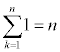1 + 1 + 1 + ... + 1 (n times)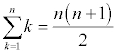1 + 2 + 3 + ... + n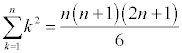1 + 4 + 9 + ... + n2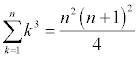1 + 8 + 27 + ... + n3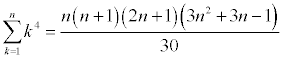1 + 16 + 81 + ... + n4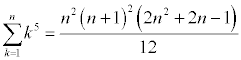1 + 32 + 243 + ... + n5

The closed form for a summation is a formula that allows you to find the sum simply by knowing the number of terms.

## Finding Closed Form

Find the sum of : 1 + 8 + 22 + 42 + ... + (3n2-n-2)

The general term is an = 3n2-n-2, so what we're trying to find is ∑(3k2-k-2), where the ∑ is really the sum from k=1 to n, I'm just not writing those here to make it more accessible.

Take the original, open form of the summation, ∑(3k2-k-2)

Distribute the summation sign, ∑3k2 - ∑k - ∑2

Factor out any constants, 3∑k2 - ∑k - 2∑1

Replace each summation by the closed form given above. The closed form is a formula for a sum that doesn't include the summation sign, only n.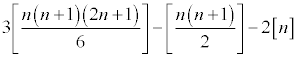Now get a common denominator, in this case, 2.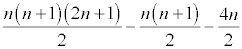Remember that the word factor begins with the letter F and anytime you have a choice of doing something in mathematics that starts with the letter F, that's probably where you should start. So, do not expand, factor instead.

The common factor is n so we'll factor that out of each term. The whole expression is over 2.

n [ (n+1)(2n+1) - (n+1) - 4 ] / 2

Now expand inside the brackets [ ].

n [ 2n2 + 3n + 1 - n - 1 - 4 ] / 2

Simplify like terms.

n ( 2n2 + 2n - 4 ) / 2

Notice the common factor of 2 inside the parentheses, let's factor that out.

2 n ( n2 + n - 2 ) / 2

The 2 in the numerator and the 2 in the denominator divide out and we can factor the rest to get the closed form for the sum.

n ( n + 2 ) ( n - 1)

Isn't that beautiful? At this point, we could write a mathematical induction problem similar to those in the book for this problem. It would read ...

Prove: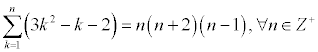We're not going to prove that statement, but that is how the book came up with many of the problems that you're asked to prove. You take an open form, find the closed form, and then put it in the text as a problem for the student to prove.

## Pattern Recognition

Sometimes you have to figure out what the general term of a sequence is. Here are some guidelines.

1. Calculate the first several terms of the sequence. Sometimes it helps to write the term in factored and expanded form.
2. Try to find a recognizable pattern. Here are some things to look for
1. Linear patterns: an + b (will have a common difference)
2. Quadratic pattern: an2 + b (the perfect squares plus/minus a constant)
3. Cubic pattern: an3 + b (the perfect cubes plus/minus a constant)
4. Exponential patterns: 2n + b, 3n + b (powers of 2 or 3 plus/minus a constant)
5. Factorial patterns: n!, (2n)!, (2n-1)! (factoring these really helps)
3. After you have your pattern, then you can use mathematical induction to prove the conjecture is correct.

## Finite Differences

Finite differences can help you find the pattern if you have a polynomial sequence.

The first differences are found by subtracting consecutive terms. If the first differences are all the same, then the pattern is linear.

The second differences are found by subtracting consecutive first differences. If the second differences are all the same, then the pattern is quadratic. Remember that you can find a quadratic model by taking the equation y=ax2+bx+c with three points. Then solve the system of equations that results. The analogy here is that you can find an=an2+bn+c by substituting in three terms in the sequence for an and their corresponding position in the sequence for n. Then solve the system of linear equations.

This can be extended to third differences by subtracting consecutive second differences. If the third differences are all the same, the pattern is cubic. You can fit a cubic model with four points and the model an=an3+bn2+cn+d.

### Finite Differences Example:

Find the general term of the sequence 1, -2, -1, 4, 13, 26, 43, 64, 89, ...

The first finite differences are found by subtracting consecutive terms in the original sequnce. That is, take -2-1=-3, -1-(-2)=1, 4-(-1)=5, 13-4=9, 26-13=13, etc.

The first finite differences are: -3, 1, 5, 9, 13, 17, 21, 25, ...

Since these aren't all the same, your sequence is not a linear (first degree) polynomial.

Move on to the second finite differences. These are the differences in the consecutive terms of the first finite differences. 1-(-3)=4, 5-1=4, 9-5=4, 13-9=4, 17-13=4, etc.

The second finite differences are 4, 4, 4, 4, 4, 4, 4, ...

Since the second finite differences are all the same, your sequence is that of a second degree polynomial and you can write the general term as an = An2 + Bn + C.

Plug in the values 1, 2, 3 (since there are three variables) into the expression.

1. When n=1, 1A + 1B + 1C = 1 (the first term is 1)
2. When n=2, 4A + 2B + 1C = -2 (the second term is -2)
3. When n=3, 9A + 3B + 1C = -1 (the third term is -1)

Now, solve that system of linear equations (I recommend using matrix inverses AX=B, so X=A-1B). If you need a refresher on how to do that, visit the section on matrix inverses in chapter 6.

In our case, we get A=2, B=-9, and C=8, so the general term of our sequence is an=2n2-9n+8.

If you want to check it, pick any value for n and plug it into the general term. You should get the nth number in the sequence. For example, if n = 6, then a6 = 2(6)2 - 9(6) + 8 = 26. Check the sequence, sure enough, the 6th number is 26.